# Basic Geometrical Ideas Exercise 4.6 Solutions Class 6 Ncert

Ncert textbook Mathematics Class 6 Chapter 4 Basic Geometrical Ideas exercise 4.6 solutions are given.

Study the lesson Basic Geometrical Ideas in the texbook.

Observe the problems and solutions given in the text book.

Observe the given below problems and solutions and try them in your own method.

You can see Solutions For Maths Class 6

Chapter 1 Knowing our Numbers

Chapter 2 whole Numbers

Chapter 3 Playing with Numbers

Chapter 4 Basic Geometrical Ideas

Exercise 4.3

Exercise 4.4

Exercise 4.5

Exercise 4.6

Chapter 6 Integers

Chapter 14 Practical Geometry

Exercise 14.1

Exercise 14.2

Exercise 14.3

Exercise 14.4

# Ncert Maths Solutions for Basic Geometrical Ideas Solutions exercise 4.6

Chapter 6 Basic Geometrical Ideas class 6

Exercise 4.6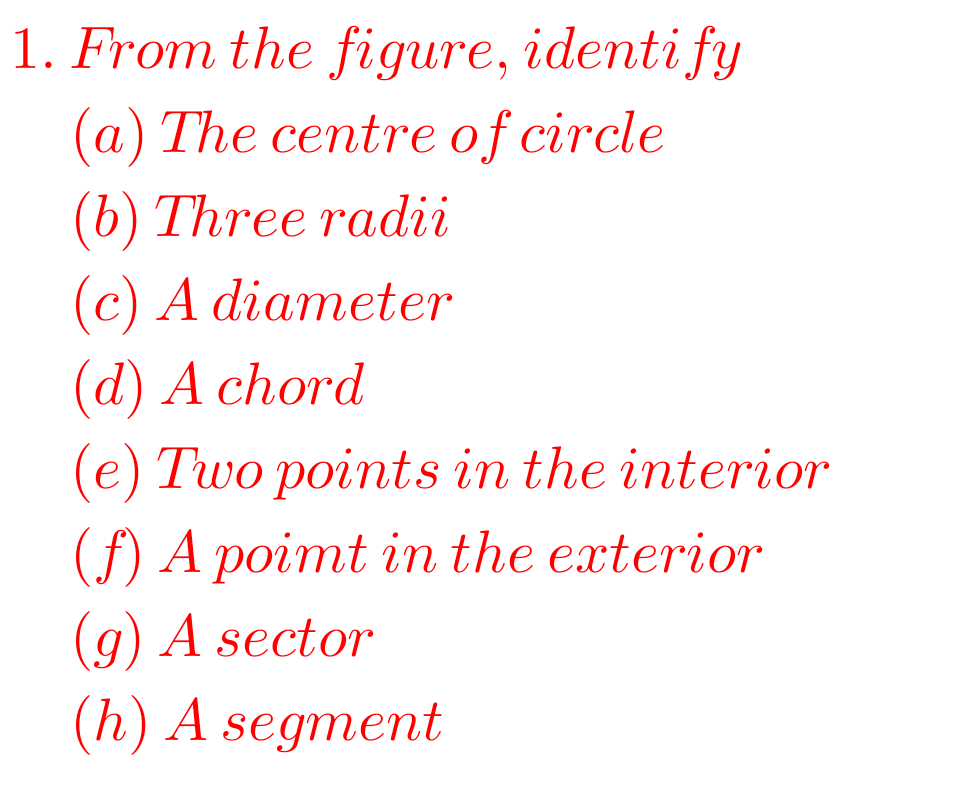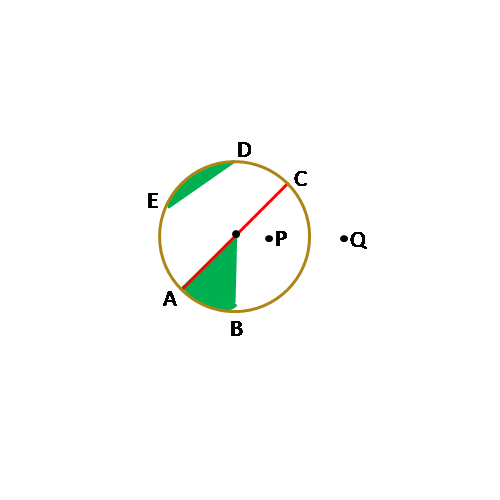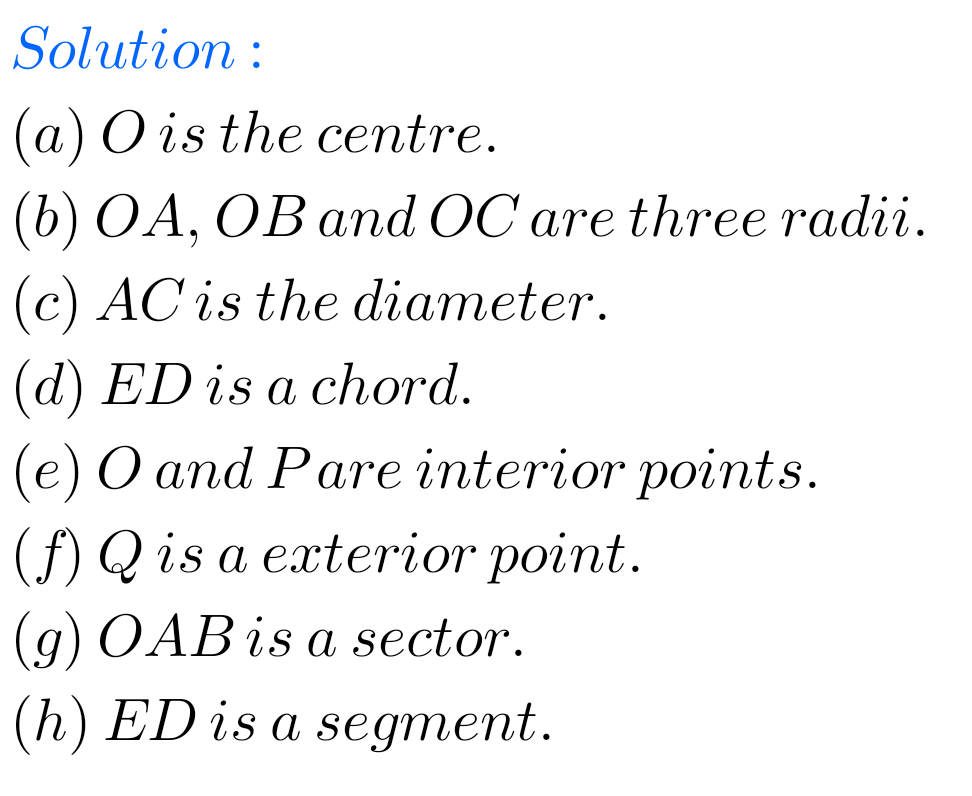## Maths Ncert Class 6th Chapter 4 exercise 4.6 solutions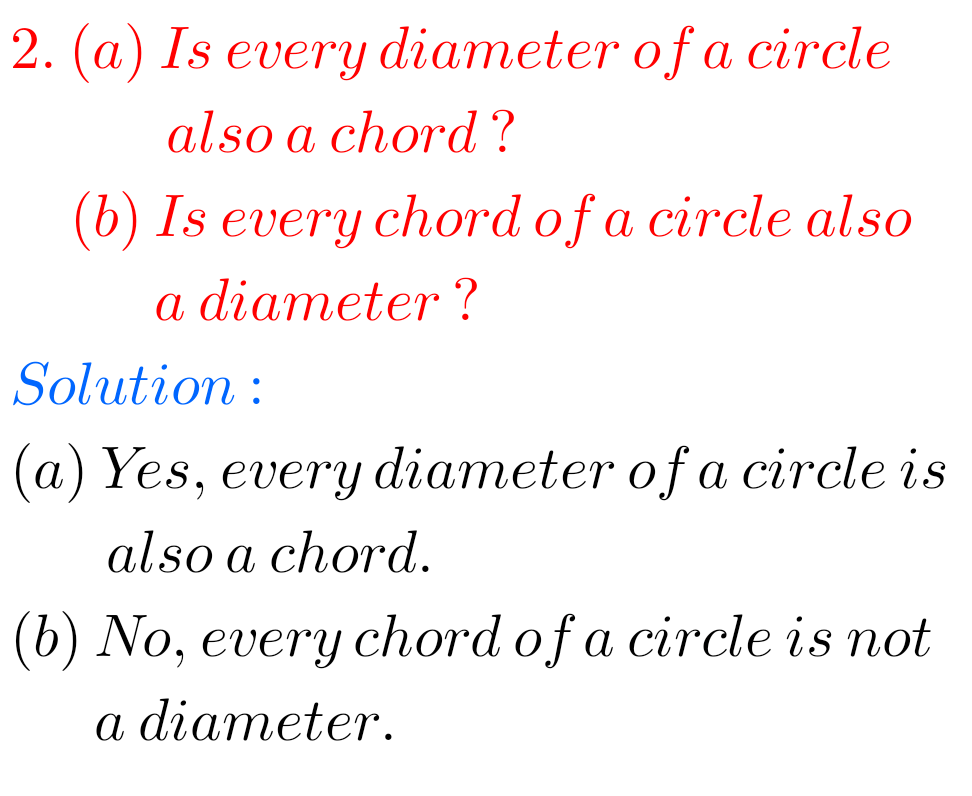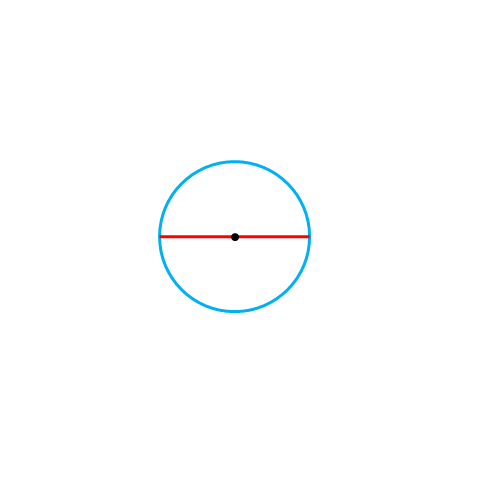## 6th class maths Basic Geometrical Ideas Exercise 4.6 Solutions Ncert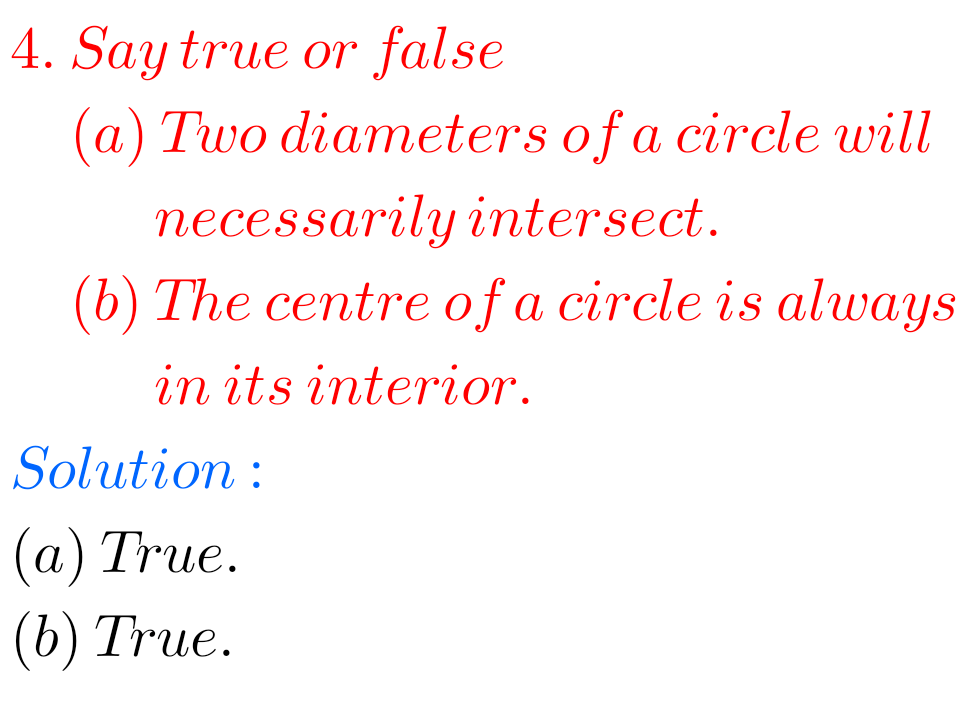Note : Observe the solutions and try them in your own method